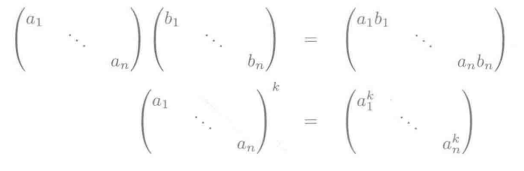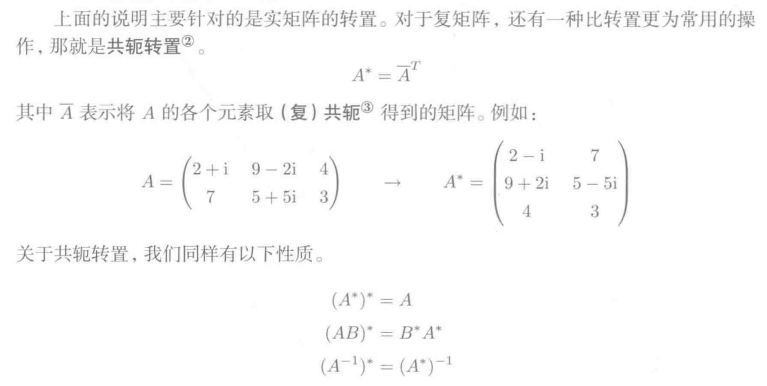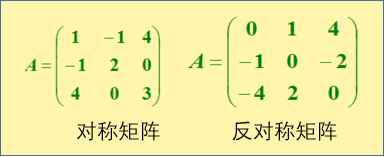• 今天小编就为大家分享一篇numpy创建单位矩阵和对角矩阵的实例，具有很好的参考价值，希望对大家有所帮助。一起跟随小编过来看看吧
• 我们已经见过一种特殊的对角矩阵单位矩阵。 不是所有的对角矩阵都是方阵，长方形的矩阵也有可能是对角矩阵。 对称矩阵 对称矩阵是转置矩阵和自己相等的矩阵： A=AT A = A^T A=AT 当某些不依赖参数顺序的双参数函数...
对角矩阵
对角矩阵只在对角线上含有非0元素，其它位置都为0。我门用

d

i

a

g

(

v

)

diag(v)

表示一个对角元素由向量

v

v

组成的对角方阵。对角矩阵的乘法计算效率很高。我们已经见过一种特殊的对角矩阵：单位矩阵。 不是所有的对角矩阵都是方阵，长方形的矩阵也有可能是对角矩阵。
对称矩阵
对称矩阵是转置矩阵和自己相等的矩阵：

A

=

A

T

A = A^T

当某些不依赖参数顺序的双参数函数生成元素时，对称矩阵经常会出现。例如，如果

A

A

是一个表示距离的矩阵，

A

i

,

j

A_{i,j}

表示点

i

i

到点

j

j

的距离，那么

A

i

,

j

=

A

j

,

i

A_{i,j}=A_{j,i}

。
单位向量
单位向量是指具有单位范数的向量：

∥

x

∥

2

=

1

\|x\|_2 = 1

如果

x

T

y

=

0

x^Ty = 0

，那么我们称向量

x

x

和向量

y

y

正交。如果2个向量都有非0范数，那么这2个向量之间的夹角是90度。在

R

n

R^n

中，最多有n个范数非0的向量正交。如果这些向量不仅正交，并且范数都为1，那么我们称他们是标准正交。
正交矩阵
正交矩阵是指行向量和列向量都是标准正交的方阵：

A

T

A

=

A

A

T

=

I

A^TA = AA^T = I

这意味着

A

−

1

=

A

T

A^{-1} = A^T


展开全文对称矩阵 正交矩阵
• 在学习linear regression时经常处理的数据一般多是矩阵或者n维向量的数据形式，所以必须对矩阵有一定的认识基础。 numpy中创建单位矩阵借助identity...单位数组的概念与单位矩阵相同，主对角线元素为1，其他元素均...
在学习linear regression时经常处理的数据一般多是矩阵或者n维向量的数据形式，所以必须对矩阵有一定的认识基础。 numpy中创建单位矩阵借助identity（）函数。更为准确的说，此函数创建的是一个n*n的单位数组，返回值的dtype=array数据形式。其中接受的参数有两个，第一个是n值大小，第二个为数据类型，一般为浮点型。单位数组的概念与单位矩阵相同，主对角线元素为1，其他元素均为零，等同于单位1。而要想得到单位矩阵，只要用mat（）函数将数组转换为矩阵即可。
>>> import numpy as np
>>> help(np.identity)

Help on function identity in module numpy:

identity(n, dtype=None)
Return the identity array.

The identity array is a square array with ones on
the main diagonal.

Parameters
----------
n : int
Number of rows (and columns) in n x n output.
dtype : data-type, optional
Data-type of the output.  Defaults to float.

Returns
-------
out : ndarray
n x n array with its main diagonal set to one,
and all other elements 0.

Examples
--------
>>> np.identity(3)
array([[ 1.,  0.,  0.],
[ 0.,  1.,  0.],
[ 0.,  0.,  1.]])
>>> np.identity(5)

array([[1., 0., 0., 0., 0.],
[0., 1., 0., 0., 0.],
[0., 0., 1., 0., 0.],
[0., 0., 0., 1., 0.],
[0., 0., 0., 0., 1.]])
>>> A = np.mat(np.identity(5))

>>> A

matrix([[1., 0., 0., 0., 0.],
[0., 1., 0., 0., 0.],
[0., 0., 1., 0., 0.],
[0., 0., 0., 1., 0.],
[0., 0., 0., 0., 1.]])

矩阵的运算中还经常使用对角阵，numpy中的对角阵用eye（）函数来创建。eye（）函数接受五个参数，返回一个单位数组。第一个和第二个参数N，M分别对应表示创建数组的行数和列数，当然当你只设定一个值时，就默认了N=M。第三个参数k是对角线指数，跟diagonal中的offset参数是一样的，默认值为0，就是主对角线的方向，上三角方向为正，下三角方向为负，可以取-n到+m的范围。第四个参数是dtype，用于指定元素的数据类型，第五个参数是order，用于排序，有‘C’和‘F’两个参数，默认值为‘C’，为行排序，‘F’为列排序。返回值为一个单位数组。
>>> help(np.eye)

Help on function eye in module numpy:

eye(N, M=None, k=0, dtype=<class 'float'>, order='C')
Return a 2-D array with ones on the diagonal and zeros elsewhere.

Parameters
----------
N : int
Number of rows in the output.
M : int, optional
Number of columns in the output. If None, defaults to N.
k : int, optional
Index of the diagonal: 0 (the default) refers to the main diagonal,
a positive value refers to an upper diagonal, and a negative value
to a lower diagonal.
dtype : data-type, optional
Data-type of the returned array.
order : {'C', 'F'}, optional
Whether the output should be stored in row-major (C-style) or
column-major (Fortran-style) order in memory.

Returns
-------
I : ndarray of shape (N,M)
An array where all elements are equal to zero, except for the k-th
diagonal, whose values are equal to one.

--------
identity : (almost) equivalent function
diag : diagonal 2-D array from a 1-D array specified by the user.

Examples
--------
>>> np.eye(2, dtype=int)
array([[1, 0],
[0, 1]])
>>> np.eye(3, k=1)
array([[ 0.,  1.,  0.],
[ 0.,  0.,  1.],
[ 0.,  0.,  0.]])

numpy中的diagonal（）方法可以对n*n的数组和方阵取对角线上的元素，diagonal（）接受三个参数。第一个offset参数是主对角线的方向，默认值为0是主对角线，上三角方向为正，下三角方向为负，可以取-n到+n的范围。第二个参数和第三个参数是在数组大于2维时指定一个2维数组时使用，默认值axis1=0，axis2=1。
>>> help(A.diagonal)

Help on built-in function diagonal:

diagonal(...) method of numpy.matrix instance
a.diagonal(offset=0, axis1=0, axis2=1)

Return specified diagonals. In NumPy 1.9 the returned array is a
read-only view instead of a copy as in previous NumPy versions.  In
a future version the read-only restriction will be removed.

Refer to :func:numpy.diagonal for full documentation.

--------
numpy.diagonal : equivalent function
>>> help(np.diagonal)

Help on function diagonal in module numpy:

diagonal(a, offset=0, axis1=0, axis2=1)
Return specified diagonals.

If a is 2-D, returns the diagonal of a with the given offset,
i.e., the collection of elements of the form a[i, i+offset].  If
a has more than two dimensions, then the axes specified by axis1
and axis2 are used to determine the 2-D sub-array whose diagonal is
returned.  The shape of the resulting array can be determined by
removing axis1 and axis2 and appending an index to the right equal
to the size of the resulting diagonals.

In versions of NumPy prior to 1.7, this function always returned a new,
independent array containing a copy of the values in the diagonal.

In NumPy 1.7 and 1.8, it continues to return a copy of the diagonal,
but depending on this fact is deprecated. Writing to the resulting
array continues to work as it used to, but a FutureWarning is issued.

Starting in NumPy 1.9 it returns a read-only view on the original array.
Attempting to write to the resulting array will produce an error.

In some future release, it will return a read/write view and writing to
the returned array will alter your original array.  The returned array
will have the same type as the input array.

If you don't write to the array returned by this function, then you can
just ignore all of the above.

If you depend on the current behavior, then we suggest copying the
returned array explicitly, i.e., use np.diagonal(a).copy() instead
of just np.diagonal(a). This will work with both past and future
versions of NumPy.

Parameters
----------
a : array_like
Array from which the diagonals are taken.
offset : int, optional
Offset of the diagonal from the main diagonal.  Can be positive or
negative.  Defaults to main diagonal (0).
axis1 : int, optional
Axis to be used as the first axis of the 2-D sub-arrays from which
the diagonals should be taken.  Defaults to first axis (0).
axis2 : int, optional
Axis to be used as the second axis of the 2-D sub-arrays from
which the diagonals should be taken. Defaults to second axis (1).

Returns
-------
array_of_diagonals : ndarray
If a is 2-D, then a 1-D array containing the diagonal and of the
same type as a is returned unless a is a matrix, in which case
a 1-D array rather than a (2-D) matrix is returned in order to
maintain backward compatibility.

If a.ndim > 2, then the dimensions specified by axis1 and axis2
are removed, and a new axis inserted at the end corresponding to the
diagonal.

Raises
------
ValueError
If the dimension of a is less than 2.

--------
diag : MATLAB work-a-like for 1-D and 2-D arrays.
diagflat : Create diagonal arrays.
trace : Sum along diagonals.

Examples
--------
>>> a = np.arange(4).reshape(2,2)
>>> a
array([[0, 1],
[2, 3]])
>>> a.diagonal()
array([0, 3])
>>> a.diagonal(1)
array()

A 3-D example:

>>> a = np.arange(8).reshape(2,2,2); a
array([[[0, 1],
[2, 3]],
[[4, 5],
[6, 7]]])
>>> a.diagonal(0, # Main diagonals of two arrays created by skipping
...            0, # across the outer(left)-most axis last and
...            1) # the "middle" (row) axis first.
array([[0, 6],
[1, 7]])

The sub-arrays whose main diagonals we just obtained; note that each
corresponds to fixing the right-most (column) axis, and that the
diagonals are "packed" in rows.

>>> a[:,:,0] # main diagonal is [0 6]
array([[0, 2],
[4, 6]])
>>> a[:,:,1] # main diagonal is [1 7]
array([[1, 3],
[5, 7]])
>>> A = np.random.randint(low=5, high=30, size=(5, 5))

>>> A

array([[25, 15, 26,  6, 22],
[27, 14, 22, 16, 21],
[22, 17, 10, 14, 25],
[11,  9, 27, 20,  6],
[24, 19, 19, 26, 14]])
>>> A.diagonal()

array([25, 14, 10, 20, 14])
>>> A.diagonal(offset=1)

array([15, 22, 14,  6])
>>> A.diagonal(offset=-2)

array([22,  9, 19])

展开全文• R语言产生各种类型的矩阵矩阵运算R语言产生一般的矩阵R语言产生单位阵R语言产生次对角阵R语言矩阵的常见运算 R语言产生一般的矩阵 # 依行排列，产生3行5列的矩阵 A = matrix(c(1:15),3,5,byrow=T) R语言产生单位...


R语言产生各种类型的矩阵及矩阵运算
R语言产生一般的矩阵R语言产生单位阵R语言产生次对角阵R语言矩阵的常见运算

R语言产生一般的矩阵
# 依行排列，产生3行5列的矩阵
A = matrix(c(1:15),3,5,byrow=T)R语言产生单位阵
#产生对角线元素为1的6x6的单位阵
A = diag(6)
#产生对角线元素为5的6x6的单位阵
A = diag(6)*5
#产对角线元素为1：6的6x6的对角阵
B = diag(c(1:6))R语言产生次对角阵
#产生次对角线元素为1的6x6的矩阵阵
A = diag(6)*0
diag(A[-1,-6]) = 1#使第一行和最后一列去掉后的方阵的对角线为1

注：思路是为去掉某行某列的矩阵对角线赋值R语言矩阵的常见运算
A = matrix(c(1:16),4,4,byrow=T);B = A
# 提取对角线元素、提取部分行，列，删除部分行列
diag(A)
A[1,];A[,2]
A[-1,];A[,-2]
# 提取下（上）三角矩阵的元素
A[lower.tri(A)]
A[upper.tri(A)]
# 矩阵四则运算
A+B					#加法
A-B					#减法
3*B					#数乘
A%*%B				#矩阵相乘
solve()			    #求逆函数

矩阵的运算还有很多需要我们大家一起探索！！！
展开全文线性代数 数据挖掘
• 矩阵矩阵就是所有元素都是0的矩阵，一般记做O。可以在后面加 m,n表示其规模。 在前一章，我们讲到，矩阵就是映射。零矩阵，就表示了将所有的点都映到原点的映射...在一个方阵中，如果从左上到右下的对角元素...
零矩阵
零矩阵就是所有元素都是0的矩阵，一般记做O。可以在后面加 m,n 表示其规模。
在前一章，我们讲到，矩阵就是映射。零矩阵，就表示了将所有的点都映到原点的映射。
因此，对于任意向量 x，都有 Ox = o。对于任意矩阵 A，都有：
A + O = O + A = A  AO = OA = O  0A = O
单位矩阵
在一个方阵中，如果从左上到右下的对角元素均为1，其余元素均为0，那么该矩阵被称为单位矩阵，常用 I 表示，用In来表示n阶单位矩阵。单位矩阵，是一个“什么都不做”的映射，对于任意向量 x，都有 Ix = x。对于任意矩阵 A，都有：AI = IA = A。
对角矩阵
在方阵中，从左上向右下方向的对角线上的值称为对角元素，其他元素称为非对角元素。非对角元素全部为0的矩阵就是对角矩阵。对角矩阵也可以用 diag( a1,a2,a3...an ) 来表示，其中diag就代表diagonal，即对角线。
对角矩阵表示的映射是“沿着坐标轴伸缩”，其中对角元素就是各轴伸缩的倍率，因此不难反过头理解单位矩阵“什么都不干”的映射。
对角矩阵的乘法和乘方规则也非常简单：逆矩阵
对于方阵A，它的逆映射对应的矩阵称为 A 的逆矩阵，记为。直接一点说，A 是将出发点 x 转换至目标点 y 的映射，而 A 的逆矩阵，则是将目标点 y 转换至出发点 x 的映射。因此，，因此，逆矩阵的定义也可以是，与 A 的乘积是单位矩阵 I 的矩阵就是 A 的逆矩阵。逆矩阵有以下这些有趣的性质：

其中，需要注意映射的前后顺序。
在这里要注意，逆矩阵并不是一定存在的。比如，将向量扁平化为一点的映射所对应的逆矩阵就是不存在的。
转置矩阵
对于矩阵 A，将其行列互换得到的矩阵，就称为 A 的转置矩阵，记为，其中 T 代表Transpose。
转置运算与乘方运算同级别，比如实际上是的意思。转置的性质主要有：

还有一点点关于共轭转置的内容，不想打了，就这么看吧：对称矩阵
设为方阵，如果满足，也就是说，，则称为对称矩阵。对称矩阵的元素以主对角线为对称轴。
类似的，如果方阵满足，即 ，则称为反对称矩阵，显然，反对称矩阵的主对角元都是零。对称矩阵也有一些有趣的规则：
对于n阶矩阵A，是对称矩阵，是反对称矩阵；  对于任意n阶矩阵A，都可以表示为对称矩阵与反对称矩阵之和  对称矩阵的和是对称矩阵，对称矩阵的乘积不一定是对称矩阵。

参考：
《程序员的数学3》
https://jingyan.baidu.com/article/da1091fb69f0b7027849d612.html

展开全文对称矩阵 转置矩阵
•线性代数
• ## 对角矩阵

千次阅读 2018-03-26 11:18:58
对角矩阵(diagonal matrix)是一个主对角线之外的元素皆为0的矩阵，常写为diag（a1，a2,...,an) 。...对角矩阵的运算包括、差运算、数乘运算、同阶对角的乘积运算，且结果仍为对角。定义 （1...
• matlab : R2018a 64bitOS : Windows 10 x64typesetting : Markdownblog : my.oschina.net/zhichengjiu创建N阶数量矩阵codeclearclceye(3)*3% 几阶乘几resultans =3 0 00 3 00 0 3>...创建N阶单位矩阵codec...
• Note：PCA主成分分析用到实对称的相似对角化。1.对角阵概念2.矩阵对角阵相似的条件3.一般矩阵的相似对角化4.实对称矩阵的相似对角化5.协方差矩阵的相似对角化(end)...
• 一般矩阵的相似对角化用它的特征向量组成的矩阵就可以了，为什么实对称矩阵的相似对角化这么特殊呢，名称叫做正交矩阵化，求得特征向量矩阵后还要正交化和单位化使之成为正交矩阵呢？ 答： 对称矩阵也可以用一般的由...
• 您为该函数提供了三个3D阵列,3D阵列的每一层代表一个主要,次要或超对角的块. (这意味着块必须具有相同的大小.)结果将是一个稀疏矩阵,因此它在内存方面应该是相当有效的.一个示例用法是：As = bsxfun(@times,ones(3),...
• 对角矩阵：M是一个对角矩阵，当且仅当i!=l时，M(i,j)=0 三对角矩阵：M是一个三对角矩阵，当且仅当|i-j|>1时，M(i,j)=0 下三角矩阵：M是一个下三角矩阵，当且仅当i<j时，M(i,j)=0 上三角矩阵：M是一个上...特殊矩阵 对三角矩阵 三角矩阵 对称矩阵
• 单位矩阵：主对角线上的元素都为1的对角矩阵。 1. 提取矩阵的对角线元素(得到一个一维矩阵) diag(A)：提取矩阵A主对角线元素，产生一个列向量。 diag(A,k)：提取矩阵A第k条对角线的元素，产生一个列向量。 >>...matlab 线性代数
• 创建对角矩阵 code clear clc a=[1,2,3]; diag(a) diag(a,1) % 上移一个单位 diag(a,2) % 上移两个单位 diag(a,-1) % 下移一个单位 result ans = 1 0 0 0 2 0 0 0 3 ans = 0 1 0 0 0 0 2 0 0 0...
• 问题描述 如题所述 解决方案 #define SIZE 3 int eye[SIZE][SIZE] = {0}; int main() { for (int i = 0; i < SIZE ; ++i) { eye[i][i] = 1;...Initialize an “eye” (identity) matrix array in CC++
• #include<stdio.h> int main() { int i,j,N,t=0,n,m,o,p=0; printf("请输入矩阵阶数N："); scanf("%d",&...A: printf("请输入对角矩阵的带宽n："); scanf("%d",&n); if(n%2==0) { pri...数据结构 C/C++ 课后作业
• （这两个最不特殊了，线代中学过不过有点忘）1、对角矩阵对角矩阵(diagonal matrix)是一个主对角线之外的元素皆为0的矩阵，常...对角线上元素全为1的对角矩阵称为单位矩阵对角矩阵的运算包括、差运算、数乘...
• 引入对角矩阵 D ==> 综上 A = L * D * U 举例 A 执行高斯消元法 ==> L 矩阵 ==> 由于，第二行没有主元，交换两行 ==> 交换过后 ==> L矩阵需要引入新的矩阵 ==> 综上 A ==线性代数
• 其中A为对角矩阵，P为单位正交。对于一般的方阵而言，不一定能对角化，对于对称矩阵而言，必能对角化，而且对于正定矩阵有λ\lambdaλi>0。 设A=[λ1λ2λn]\left[\begin{matrix} \lambda_1& &\\&\...
• 文章目录相似矩阵矩阵对角化对称矩阵对角化对称A对角化的步骤 相似矩阵 设A，B都是n阶矩阵，若有可逆矩阵P，使 P−1AP=B,P^{-1}AP=B,P−1AP=B,则称B是A的相似矩阵，或说矩阵A与B相似，对A进行运算P−1APP^{-1...线性代数
• 从键盘输入矩阵的行数列数，随机生成稀疏矩阵。 设计算法将随机生成的稀疏矩阵转换成三元组顺序表形式存储。 设计算法将快速装置得到的与相加得到的三元组顺序表分别转换成矩阵形式。 输出随机生成的稀疏矩阵A...
• - [矩阵对角化](#矩阵对角化) - [SVD分解](#svd分解) - [参考链接](#参考链接) 矩阵对角矩阵的相似 设 A\boldsymbol{A}A、 B\boldsymbol{B}B 为两个nnn阶矩阵，若存在可逆矩阵 P\boldsymbol{P}P，使得...线性代数 SVD
• 详细的代码可见github： ... 对角矩阵(diagonal matrix)是一个主对角线之外的元素皆为0的矩阵，...对角矩阵可以认为是矩阵中最简单的一种，值得一提的是：对角线上的元素可以为 0 或其他值，对角线上元素相等的对角矩...数据结构
• ## 矩阵对角化

万次阅读 2015-10-16 16:52:47
一个映射或者一个线性变换，都有一个矩阵和它相对应。矩阵或者映射是不是可以对角化，对工程应用来说比较重要，因为对角化后的矩阵，乘积简单，经过多次变换的话，相当于矩阵的多次方。矩阵能不能对角化，取决于它...
• ## 单位矩阵和逆矩阵

千次阅读 2018-11-20 16:13:00
任意向量和单位矩阵相乘，都不会改变 　我们将保持 n 维向量不变的单位矩阵记作 I n ，形式上，I n ∈ R n×n 2.矩阵的逆　 　矩阵 A 的矩阵逆（matrix inversion）记作 A −1 ，其定义的矩阵满足如下条件 ......